JEE  >  Competition Level Test: Continuity And Differentiability- 3

# Competition Level Test: Continuity And Differentiability- 3

Test Description

## 13 Questions MCQ Test Mathematics (Maths) Class 12 | Competition Level Test: Continuity And Differentiability- 3

Competition Level Test: Continuity And Differentiability- 3 for JEE 2023 is part of Mathematics (Maths) Class 12 preparation. The Competition Level Test: Continuity And Differentiability- 3 questions and answers have been prepared according to the JEE exam syllabus.The Competition Level Test: Continuity And Differentiability- 3 MCQs are made for JEE 2023 Exam. Find important definitions, questions, notes, meanings, examples, exercises, MCQs and online tests for Competition Level Test: Continuity And Differentiability- 3 below.
Solutions of Competition Level Test: Continuity And Differentiability- 3 questions in English are available as part of our Mathematics (Maths) Class 12 for JEE & Competition Level Test: Continuity And Differentiability- 3 solutions in Hindi for Mathematics (Maths) Class 12 course. Download more important topics, notes, lectures and mock test series for JEE Exam by signing up for free. Attempt Competition Level Test: Continuity And Differentiability- 3 | 13 questions in 26 minutes | Mock test for JEE preparation | Free important questions MCQ to study Mathematics (Maths) Class 12 for JEE Exam | Download free PDF with solutions
 1 Crore+ students have signed up on EduRev. Have you?
Competition Level Test: Continuity And Differentiability- 3 - Question 1

### If f(x) =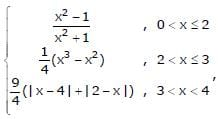, then

Competition Level Test: Continuity And Differentiability- 3 - Question 2

### The number of points at which the function f(x) = max. {a – x, a + x, b} – ∞ < x < ∞, 0 < a < b cannot be differentiable is

Detailed Solution for Competition Level Test: Continuity And Differentiability- 3 - Question 2

A function is not differentiable at the points where it has sharp-corner point
Clearly above function has two sharp corner, so it is not differentiable at these two points,
Other than these points, function linear continuous, so it is diferentiable .
Hence above function is not differentiable at two points

Competition Level Test: Continuity And Differentiability- 3 - Question 3

### The function f(x) is defined by f(x) =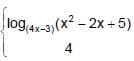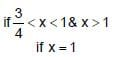Detailed Solution for Competition Level Test: Continuity And Differentiability- 3 - Question 3

lt x1^+   f(x) = log(4x -3) (x2- 2x + 5)
= ln(x2 - 2x +5)/ln(4x-3)
lt(h → 0)   ln(1+h)2 - 2(1+h) + 5)/ln(4(1+h) - 3)
lt(h → 0)   ln(1+h2 + 2h - 2 -2h + 5)/ln(4 + 4h - 3)
ln(h → 0)    ln(4 + h2)/(1+4h)
Divide and multiply the denominator by 4h
ln(h → 0)    ln(4 + h2)/[((1+4h)/4h) * 4h]
As we know that (1+4h)/4h = 1
ln 4/(4*0)   = + ∞ (does not exist)
lt x→ 1^-   f(x) = log(4x -3) (x2- 2x + 5)
lt(h → 0)   ln(1-h)2 - 2(1-h) + 5)/ln(4(1-h) - 3)
lt(h → 0)   ln(1+h2 - 2h - 2 + 2h + 5)/ln(4 - 4h - 3)
ln(h → 0)    ln(4 + h2)/(1 - 4h)
Divide and multi[ly the denominator by (-4h)
ln(h → 0)    ln(4 + h2)/[((1+4h)/-4h) * (-4h)
As we know that (1-4h)/(-4h) = 1
ln 1/(-4*0)   = - ∞ (does not exist)

Competition Level Test: Continuity And Differentiability- 3 - Question 4

Let f(x) =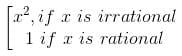then

Competition Level Test: Continuity And Differentiability- 3 - Question 5

A point where function f(x) is not continuous where f(x) = [sin [x]] in (0, 2π) ; is ([ * ] denotes greatest integer ≤ x)

Competition Level Test: Continuity And Differentiability- 3 - Question 6

Let f(x) = [n + p sin x], x ∈ (0, π), n ∈ I and p is a prime number. Then number of points where f(x) is not differentiable is (where [ * ] denotes greatest integer function)

Detailed Solution for Competition Level Test: Continuity And Differentiability- 3 - Question 6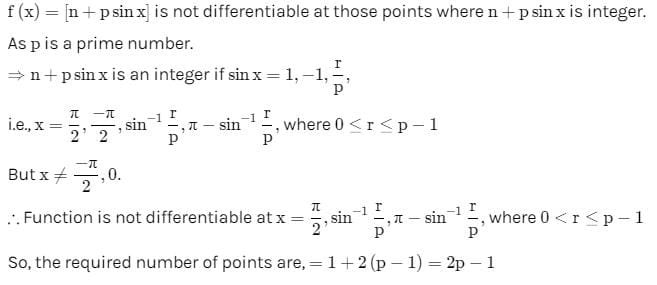Competition Level Test: Continuity And Differentiability- 3 - Question 7

If f is a real-valued differentiable function satisfying |f(x) – f(y)| ≤ (x – y)2, x, y ∈ R and f(0) = 0, then f(1) equals

Competition Level Test: Continuity And Differentiability- 3 - Question 8

If f(x) =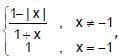, then f([2x]) is (where [ * ] represent greatest integer function)

Competition Level Test: Continuity And Differentiability- 3 - Question 9

The value of f(0), so that the function, f(x)=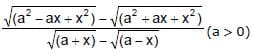becomes continuous for all x, is given by

Detailed Solution for Competition Level Test: Continuity And Differentiability- 3 - Question 9

f(x)={(a2−ax+x2)−(a2+ax+x2)^1/2}/{(a+x -(a-x)1/2)1/2}
on rationalising, we get
lom(x → 0) a2 - ax + x2 -(a2 +ax + x2) * (a+x)1/2 + (a-x)1/2]/[(a+x)-(a-x) * (a2 - ax + x2)1/2 + (a2 + ax + x2)]
lim(x → 0) [-2ax * [(a)1/2 + (a)1/2]]/[2x * (a2)1/2 * (a2)1/2]
-a/(a)1/2 = -(a)1/2

Competition Level Test: Continuity And Differentiability- 3 - Question 10

If f(x) =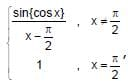then f(x) is (where { * } represents the fractional part function)

Competition Level Test: Continuity And Differentiability- 3 - Question 11

In order that function f (x) = (x + 1)cot x is continuous at x = 0, f (0) must be defined as

Competition Level Test: Continuity And Differentiability- 3 - Question 12

f is a continuous function on the real line. Given that x2 + (f(x) – 2) x –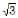. f(x) + 2– 3 = 0. then the value of f()

Competition Level Test: Continuity And Differentiability- 3 - Question 13

Given f(x)=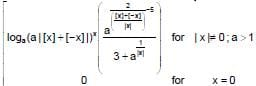then (where [*] represent the integral part function)

## Mathematics (Maths) Class 12

209 videos|218 docs|139 tests
Information about Competition Level Test: Continuity And Differentiability- 3 Page
In this test you can find the Exam questions for Competition Level Test: Continuity And Differentiability- 3 solved & explained in the simplest way possible. Besides giving Questions and answers for Competition Level Test: Continuity And Differentiability- 3, EduRev gives you an ample number of Online tests for practice

## Mathematics (Maths) Class 12

209 videos|218 docs|139 tests(Scan QR code)